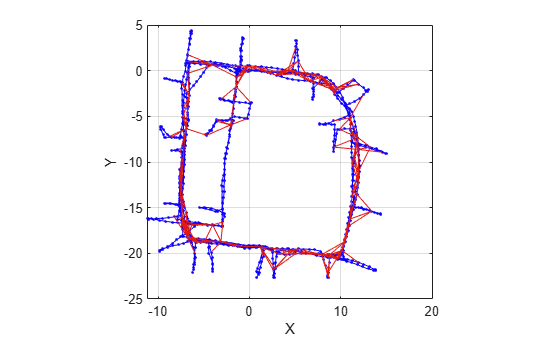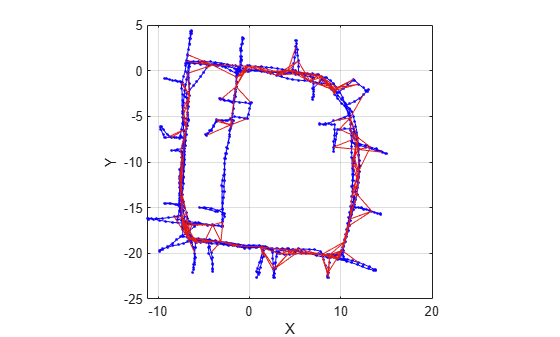# removeEdges

Remove loop closure edges from graph

Since R2019b

## Syntax

``removeEdges(poseGraph,edgeIDs)``

## Description

example

````removeEdges(poseGraph,edgeIDs)` removes loop closure edges, landmark edges, or duplicate incremental edges from the pose graph.```

## Examples

collapse all

Load the Intel Research Lab Dataset that contains a 2-D pose graph. Optimize the pose graph. Plot the pose graph with IDs off. Red lines indicate loop closures identified in the dataset.

```load intel-2d-posegraph.mat pg optimizedPG = optimizePoseGraph(pg); show(optimizedPG,IDs="off");```Find the loop closure edge ID for the specified edge node pair in pose graph.

```nodePair = [133 1085]; edgeID = findEdgeID(optimizedPG,nodePair)```
```edgeID = 1386 ```

Remove the loop closure edge from the pose graph.

```removeEdges(optimizedPG,edgeID) show(optimizedPG,IDs="off");```## Input Arguments

collapse all

Pose graph, specified as a `poseGraph` or `poseGraph3D` object.

Edge IDs, specified as a vector of positive integers. To get edge IDs based on node pairs, see the `findEdgeID` function.

## Version History

Introduced in R2019b